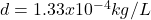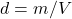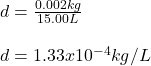## A 15.00 L sample of Helium gas has a mass of .002 kg. What is the density of this sample? Your answer should be expressed in proper

Question

A 15.00 L sample of Helium gas has a mass of .002 kg. What is the

in progress 0
2 months 2021-07-26T11:03:58+00:00 1 Answers 2 views 0Explanation:

Hello there!

In this case, according to the given information, it turns out possible for us to calculate the required density by knowing it is calculated by dividing mass over volume:Therefore, we plug in the given kilograms and liters to obtain:Which shows the proper scientific notation.

Regards!